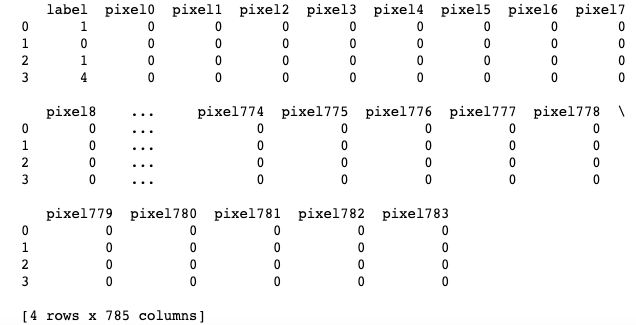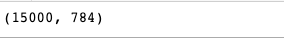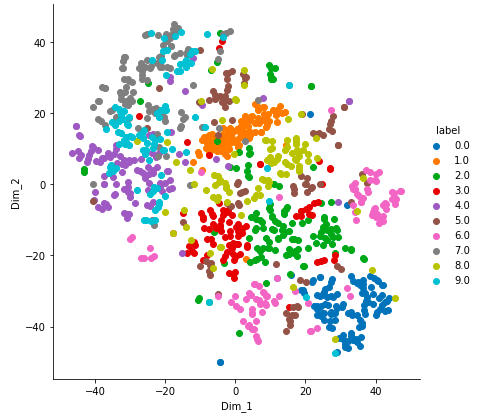# ML | T-distributed Stochastic Neighbor Embedding (t-SNE) Algorithm

T-distributed Stochastic Neighbor Embedding (t-SNE) is a nonlinear dimensionality reduction technique well-suited for embedding high-dimensional data for visualization in a low-dimensional space of two or three dimensions.

What is Dimensionality Reduction?
Dimensionality Reduction is the technique of representing n-dimensions data(multidimensional data with many features) in 2 or 3 dimensions.

An example of dimensionality reduction can be discussed as a classification problem i.e. student will play football or not that relies on both temperature and humidity can be collapsed into just one underlying feature, since both of the features are correlated to a high degree. Hence, we can reduce the number of features in such problems. A 3-D classification problem can be hard to visualize, whereas a 2-D one can be mapped to simple 2-dimensional space and a 1-D problem to a simple line.

How does t-SNE works?
t-SNE a non-linear dimensionality reduction algorithm finds patterns in the data based on the similarity of data points with features, the similarity of points is calculated as the conditional probability that a point A would choose point B as its neighbor.
It then tries to minimize the difference between these conditional probabilities (or similarities) in higher-dimensional and lower-dimensional space for a perfect representation of data points in lower-dimensional space.

Space and Time Complexity
The algorithm computes pairwise conditional probabilities and tries to minimize the sum of the difference of the probabilities in higher and lower dimensions. This involves a lot of calculations and computations. So the algorithm takes a lot of time and space to compute. t-SNE has a quadratic time and space complexity in the number of data points.

### Applying t-SNE on MNIST dataset

 `# Importing Necessary Modules. ` `import` `numpy as np ` `import` `pandas as pd ` `import` `matplotlib.pyplot as plt ` `from` `sklearn.manifold ``import` `TSNE ` `from` `sklearn.preprocessing ``import` `StandardScaler `

 `# Reading the data using pandas   ` `df ``=` `pd.read_csv(``'mnist_train.csv'``) ` ` `  `# print first five rows of df ` `print``(df.head(``4``))  ` ` `  `# save the labels into a variable l. ` `l ``=` `df[``'label'``] ` ` `  `# Drop the label feature and store the pixel data in d. ` `d ``=` `df.drop(``"label"``, axis ``=` `1``) `

Output:Code #2: Data-preprocessing

 `# Data-preprocessing: Standardizing the data ` `from` `sklearn.preprocessing ``import` `StandardScaler ` ` `  `standardized_data ``=` `StandardScaler().fit_transform(data) ` ` `  `print``(standardized_data.shape) `

Output:Code #3:

 `# TSNE ` `# Picking the top 1000 points as TSNE ` `# takes a lot of time for 15K points ` `data_1000 ``=` `standardized_data[``0``:``1000``, :] ` `labels_1000 ``=` `labels[``0``:``1000``] ` ` `  `model ``=` `TSNE(n_components ``=` `2``, random_state ``=` `0``) ` `# configuring the parameteres ` `# the number of components = 2 ` `# default perplexity = 30 ` `# default learning rate = 200 ` `# default Maximum number of iterations ` `# for the optimization = 1000 ` ` `  `tsne_data ``=` `model.fit_transform(data_1000) ` ` `  ` `  `# creating a new data frame which ` `# help us in ploting the result data ` `tsne_data ``=` `np.vstack((tsne_data.T, labels_1000)).T ` `tsne_df ``=` `pd.DataFrame(data ``=` `tsne_data, ` `     ``columns ``=``(``"Dim_1"``, ``"Dim_2"``, ``"label"``)) ` ` `  `# Ploting the result of tsne ` `sn.FacetGrid(tsne_df, hue ``=``"label"``, size ``=` `6``).``map``( ` `       ``plt.scatter, ``'Dim_1'``, ``'Dim_2'``).add_legend() ` ` `  `plt.show() `

Output:My Personal Notes arrow_drop_upCheck out this Author's contributed articles.

If you like GeeksforGeeks and would like to contribute, you can also write an article using contribute.geeksforgeeks.org or mail your article to contribute@geeksforgeeks.org. See your article appearing on the GeeksforGeeks main page and help other Geeks.

Please Improve this article if you find anything incorrect by clicking on the "Improve Article" button below.

Article Tags :
Practice Tags :

2

Please write to us at contribute@geeksforgeeks.org to report any issue with the above content.